# Civil Engineering - Soil Mechanics and Foundation Engineering

### Exercise :: Soil Mechanics and Foundation Engineering - Section 1

21.

Minimum depth of a footing carrying a heavy load, is calculated by the formula

 A.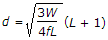B.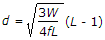C.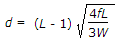D.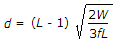Explanation:

No answer description available for this question. Let us discuss.

22.

According to Coulomb's wedge theory, the active earth pressure slides the wedge

 A. down and outwards on a slip surface B. up and inwards on a slip surface C. horizontal upward and parallel to base D. horizontal inward and parallel to base.

Explanation:

No answer description available for this question. Let us discuss.

23.

The coefficient of curvature is defined

 A.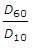B.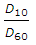C.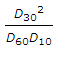D.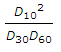Explanation:

No answer description available for this question. Let us discuss.

24.

The effective size of particles of soil is denoted by

 A. D10 B. D20 C. D30 D. D60

Explanation:

No answer description available for this question. Let us discuss.

25.

Degree of saturation of a natural soil deposit having water content 15%, specific gravity 2.50 and void ratio 0.5, is

 A. 50% B. 60% C. 75% D. 80%